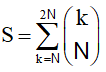David F.

# finding an sum equation - Combinatorics

I need help finding an equation for the next formula and getting rid of the SUM sign